Question

For the following transfer function, sketch approximate straight-line Bode plots, including magnitude and phase plots. Show all steps clearly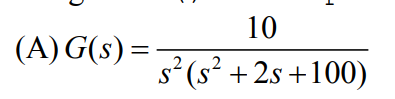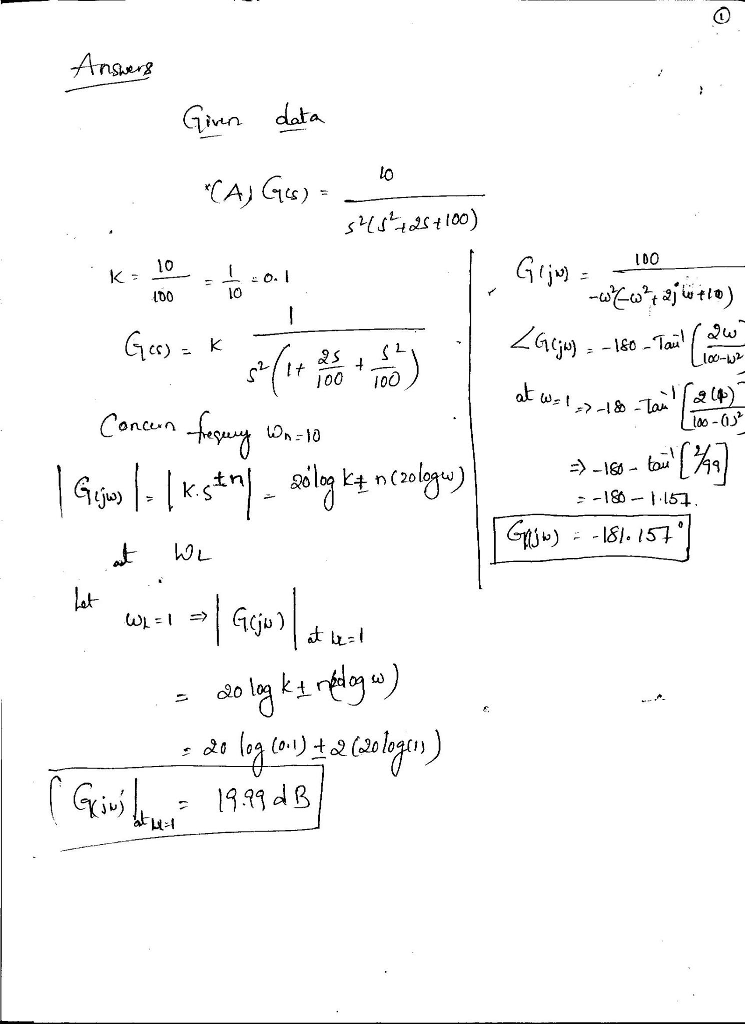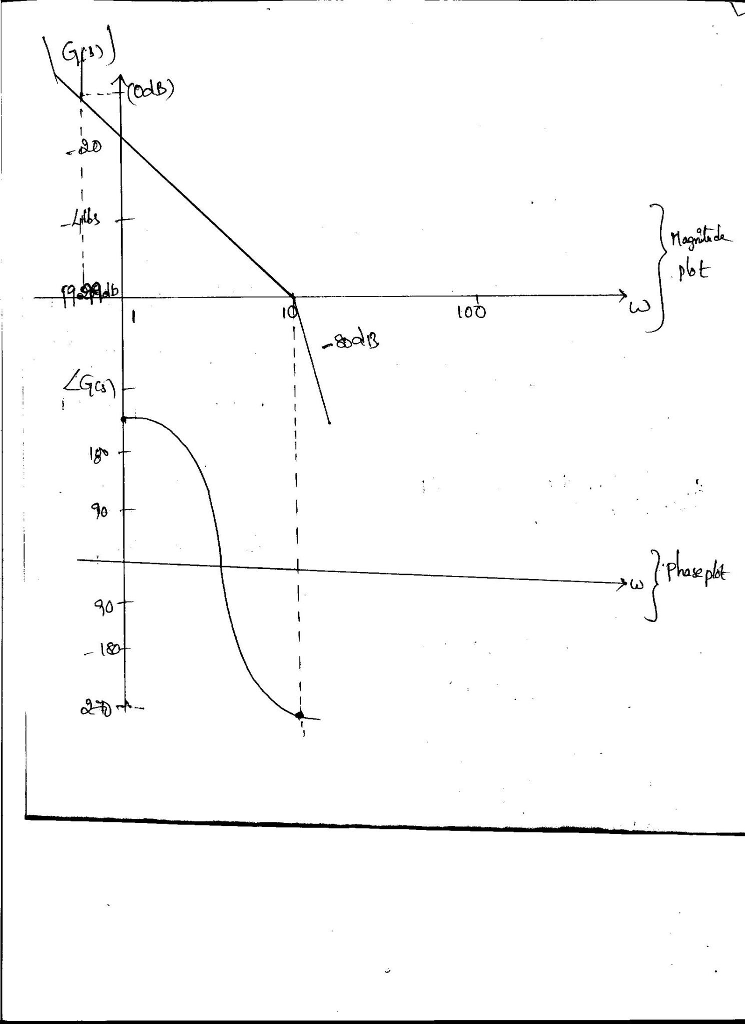#### Earn Coins

Coins can be redeemed for fabulous gifts.

Similar Homework Help Questions
• ### Sketch the approximate Bode magnitude and phase plots for the following transfer functions by hand. a....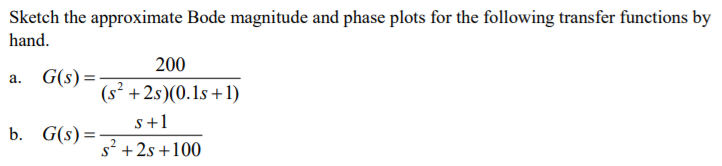Sketch the approximate Bode magnitude and phase plots for the following transfer functions by hand. a. G(s) b. G(s)- 200 (s2 +2s)(0.1s +1) s+1 s2 +2s +100

• ### Sketch the Bode magnitude and phase plots for the following transfer function: G(s)=- a fimction: G(9)=...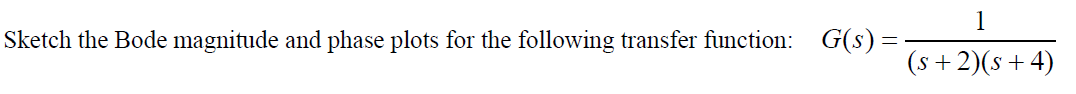Sketch the Bode magnitude and phase plots for the following transfer function: G(s)=- a fimction: G(9)= (s+2016+4) (s + 2)(+4)

• ### For the following closed-loop transfer functions, sketch the bode plots (magnitude and phase), id...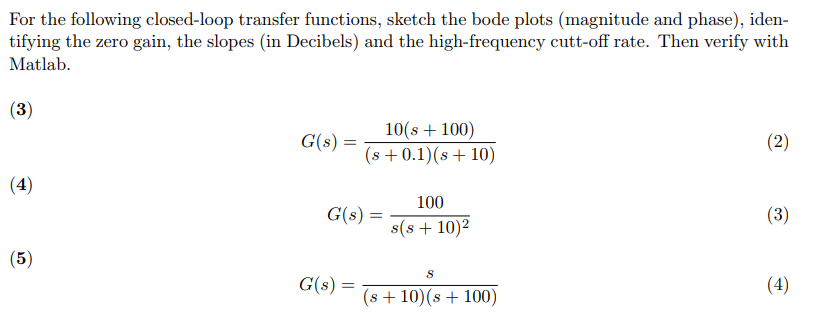For the following closed-loop transfer functions, sketch the bode plots (magnitude and phase), iden- tifying the zero gain, the slopes (in Decibels) and the high-frequency cutt-off rate. Then verify with Matlab C()101 100) s 0.1) (s 10) 100 s(s +10)2 G(s) = (56) G(s) = s+10(s+100) For the following closed-loop transfer functions, sketch the bode plots (magnitude and phase), iden- tifying the zero gain, the slopes (in Decibels) and the high-frequency cutt-off rate. Then verify with Matlab C()101 100) s...

• ### 2. Use straight line approximation to sketch bode (gain and phase) plots for 1000s G1(s) s+...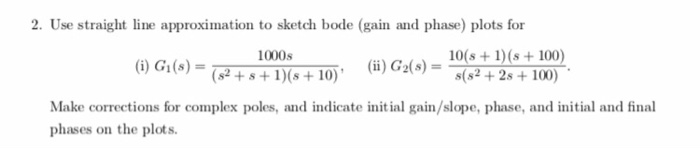2. Use straight line approximation to sketch bode (gain and phase) plots for 1000s G1(s) s+ IG+ 10 ()G)0 1 100 s(s2 +2s+100) Make corrections for complex poles, and indicate initial gain/slope, phase, and initial and final phases on the plots

• ### i) Draw the Bode plots (hand sketch, magnitude and phase!) for the following transfer function. Plot...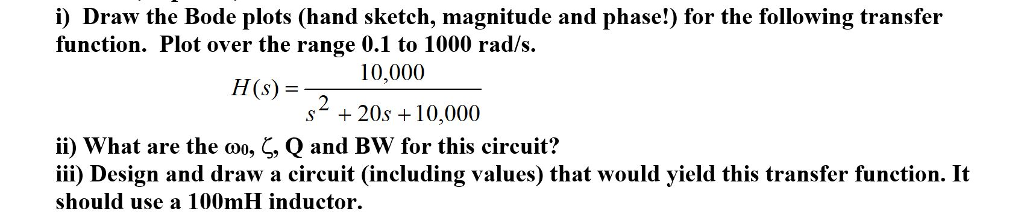i) Draw the Bode plots (hand sketch, magnitude and phase!) for the following transfer function. Plot over the range 0.1 to 1000 rad/s HS 10,000 (s) = s* + 20s 10,000 ii) what are the Q and Bw for this circuit? iii) Design and draw a circuit (including values) that would yield this transfer function. It should use a 100mH inductor , , Qano

• ### Bode Plots Sketch the Bode plot magnitude and phase for each of the three open-loop transfer...

Bode Plots Sketch the Bode plot magnitude and phase for each of the three open-loop transfer functions listed below. Verify your results using the bode m function in MATLAB.(a) $$G(s)=\frac{100}{s(0.1 s+1)(0.01 s+1)}$$(b) $$G(s)=\frac{1}{(s+1)^{2}\left(s^{2}+s+9\right)}$$(c) $$G(s)=\frac{16000 s}{(s+1)(s+100)\left(s^{2}+5 s+1600\right)}$$

• ### 2. Sketch the Bode straight line plots for magnitude and phase. a) H60) = a[i*3 (veja)...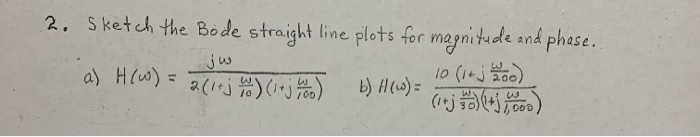2. Sketch the Bode straight line plots for magnitude and phase. a) H60) = a[i*3 (veja) b)H(s) og 10 (14 j k loo) (j +5.000) jw

• ### 1. (a) sketch the Bode magnitude and phase plots of a transfer function T(W) = Vo(w)...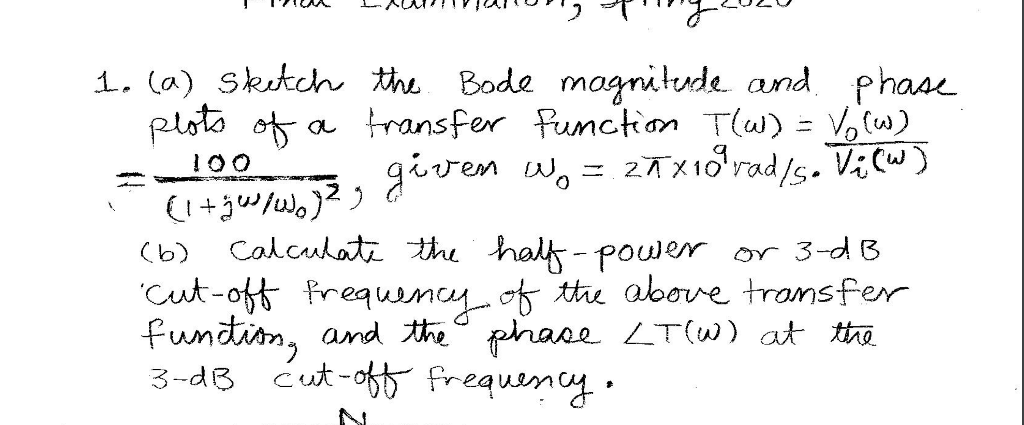1. (a) sketch the Bode magnitude and phase plots of a transfer function T(W) = Vo(w) given Wo = 27x1oʻrad/s. Vi (w) (l+ges/wo2 g (6) Calculate the half-power or 3-dB Cut-off frequency of the above transfer function, and the phase LT(W) at the 3-dB cut-off frequency.

• ### 1- For each transfer function below, sketch the Bode magnitude and phase plots, a) T(s) 3040S...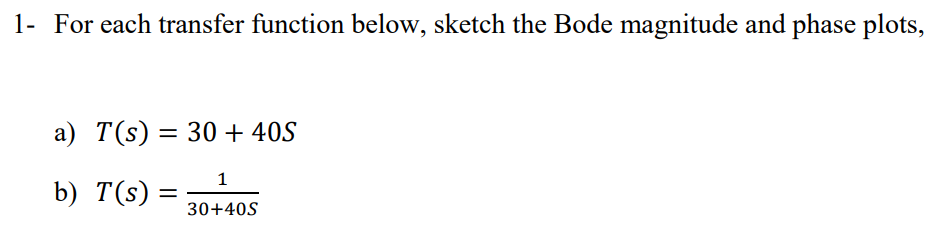1- For each transfer function below, sketch the Bode magnitude and phase plots, a) T(s) 3040S b) T(S) 30-405

• ### a) Please show all your steps. For the magnitude and phase angle plots, approximate bode diagrams...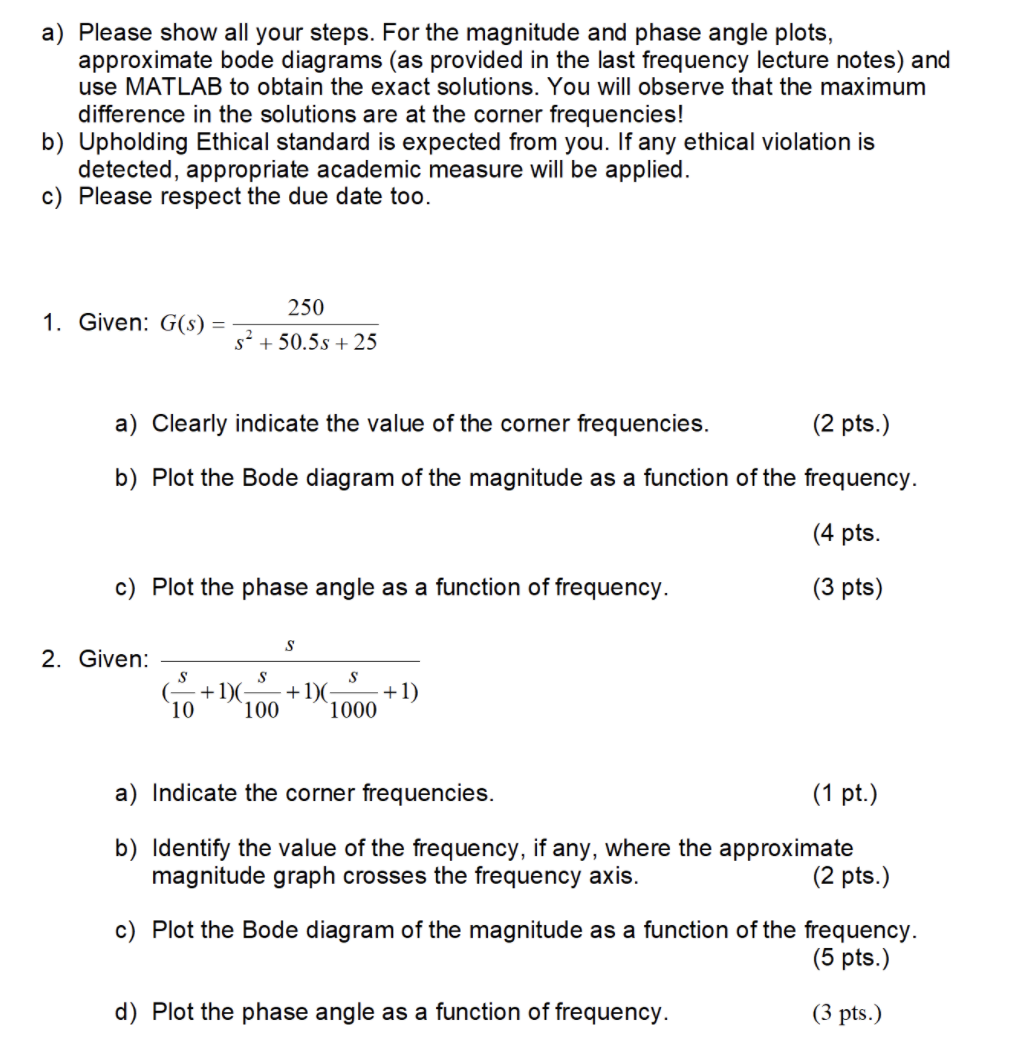a) Please show all your steps. For the magnitude and phase angle plots, approximate bode diagrams (as provided in the last frequency lecture notes) and use MATLAB to obtain the exact solutions. You will observe that the maximum difference in the solutions are at the corner frequencies! b) Upholding Ethical standard is expected from you. If any ethical violation is detected, appropriate academic measure will be applied. c) Please respect the due date too. 250 1. Given: G(S) = 52...